# Date Formula Examples – Excel & Google Sheets

This tutorial will provide an overview of date formulas in Excel & Google Sheets.

<<master image>>

## Dates Overview

In spreadsheets, dates are stored as serial numbers where each whole number represents a unique day. When you enter a date into a cell, the date is automatically converted to a serial number and the cell number formatting is changed to a Date format so the date is readable.

<<gif?>>

In Excel, the serial number 1 represents the date 1/1/1900.

You can always change the date formatting so the serial number is visible by changing the Number Format to General:

<<example or gif>>

## Creating Dates

### TODAY Function

The TODAY Function will output today’s date.

<<example>>

Keep in mind that this date will refresh every time the workbook is recalculated. To hard-code the current date use the shortcut CTRL + ;.

### DATE Function

To enter dates in formulas, you can use the DATE Function.

<<example>>

### Automatically Fill Dates

If you enter a date, and drag the date down, you can quickly populate a series of dates (although instead, you might consider using a +1 formula instead):

<<example>>

## Date Math

### Easy Excel Automation### Add or Subtract Days or Weeks to Dates

Because dates are stored as serial numbers, you can simply add (or subtract) whole numbers to dates to add (or subtract) days to a date.

<<example>>

You can also subtract one date from another date to calculate the number of days between them.

``=C3-B3``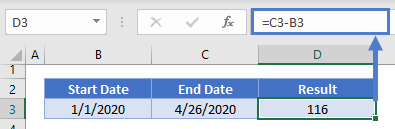To add weeks to a date, simply add the number of weeks multiplied by 7.

<<example>>

### Add or Subtract Months or Years to Dates

The EDATE Function is used to add (or subtract) months or years to a date.  This example will add months to a date:

<<example>>

To add years to a date, simply add the number of years and multiply by 12.

<<example>>

You can also add a number of business days (workdays or weekdays) to a date with the WORKDAY Function:

<<example>>

### Count Workdays Between Dates

Or calculate the number of workdays between two days with the NETWORKDAYS Function:

<<example>>

Each of these functions can also optionally include holidays:

<<example of just one of the functions>>

## Extract Information from Date

To extract the Day, Month, or Year numbers from dates use the DAY, MONTH, and YEAR Functions.

### DAY Function

The DAY Function calculates the day number of a date.

<<day example>>

### MONTH Function

The MONTH Function calculates the month number of a date (which can be used to calculate the month name as we’ll demonstrate below).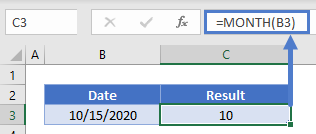### YEAR Function

The YEAR Function calculates the year number of a date.

<<Year Example>>

AutoMacro | Excel Automation Made Easy | Free Trial

### Date Function

By using the DATE Function along with the DAY, MONTH, and YEAR Functions, you can create a date similar to an existing date. This example will calculate the first day of the year from a date:

<<example of first day of year calc>>

### Quarter From a Date

If you need to calculate a date’s quarter you can use the **** :

<<example>>

### Get Month Name From Date

To calculate the month name from a date, you can simply change the Number Formatting of a date to see the month name.

<<GIF>>

Or you can use the TEXT Function to output the month name as text:

``=TEXT(B3,"mmmmm")``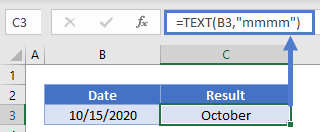### WEEKNUM

To calculate the week number of a date (1-54), use the WEEKNUM Function.

<<example>>

AutoMacro | Excel Automation Made Easy | Free Trial

### Day of Week Number / Name

The ***** Function will return the day number of a date within a week.

<<example>>

This can be adjusted to start the week on a different day (Ex. Monday instead of Sunday).

<<example>>

Then you can use the CHOOSE Function to calculate the name of the day:

<<example>>

## EOMONTH Function

Early we discussed the EDATE Function that allows you to “jump” forwards or backwards a defined number of months. The EOMONTH Function works in the same way except the EOMONTH Function will return the last day of a month:

``=EOMONTH(B3,-1)``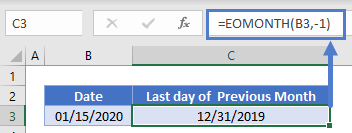By adding 1 day to the result of the EOMONTH Function you can calculate the first day of a month:

``=EOMONTH(B3,-1)+1``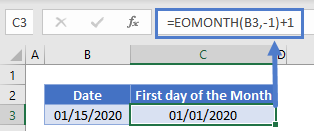## Comparing Dates

You can compare dates by using Excel’s standard comparison operators.

<<image / chart>>

<<example / look at formula page>>

## Date Formatting

Dates can be formatted in many different ways. You can refer to day, months, and years using any of the below options:

<<image>>

And combine them in a variety of ways

<<image>>

You can access these formatting options through the Format Cells menu.

***talk about how to access this…***

AutoMacro | Excel Automation Made Easy | Free Trial

### Convert Date to Text

We briefly mentioned the TEXT Function above. The TEXT Function allows you to output a number (in this case a date) as text with a specific number format.

This example will output …

<<example>>

Convert Text to Date

If you have a date stored as text, the DATEVALUE Function will convert it to a date:

<<example>>

### Date Conditional Formatting

DATEDIFF Function

misc. formulas

create date range from two dates

extract quarter from date

conditional formatting

max date

split date and times into separate cells? probably not

number of days in month

first day of month

day number of year

convert month name to number

years of service

sort by month?

concatenate date

average time, total time

### Excel Practice Worksheet

Practice Excel functions and formulas with our 100% free practice worksheets!